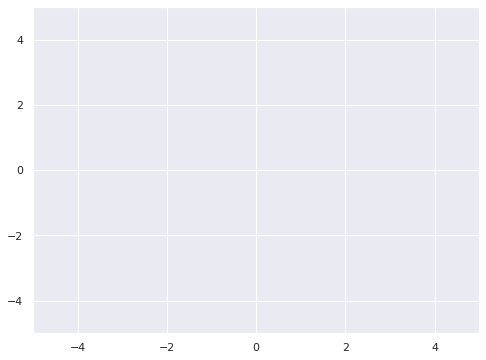# Second New Post

Write your post here. I don't want to

# First notebook post

## First Notebook Post¶

This site will provide easy to follow deep learning tutorials, mainly in pytorch.

In :
%matplotlib inline

In :
import numpy as np
import matplotlib.pyplot as plt
import seaborn as sns
sns.set()
from matplotlib import animation, rc
from IPython.display import HTML


Some basic math below:

\begin{equation} \bar{x} = \sum_{i}^{N} x_i p(x_i) \end{equation}
In :
fig, ax = plt.subplots(figsize=(8,6))
ax.set_ylim(-5, 5)
ax.set_xlim(-5, 5);
plot = ax.scatter([], [], s=1, c='r')In :
def init():
plot.set_offsets(([], []))
return plot

def animate(i):
data = np.random.multivariate_normal([0,0],[[1, (i/100)*4/5],[(i/100)*4/5, 1]], size=1000)
plot.set_offsets(data)

return plot

anim = animation.FuncAnimation(fig, animate, init_func=init, frames=100, interval=10, blit=False)

In :
HTML(anim.to_jshtml())

Out: